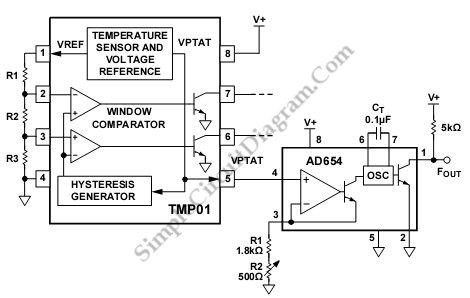# TMP01 AD654 Temperature-to-Frequency Converter

This is a TMP01 Temperature-to-Frequency Converter circuit. Converting voltage to the frequency domain is another common method of transmitting analog information. This circuit uses monolithic voltage-to-frequency converters (VFCs) that has some advantages like low cost a robust, and has open-collector digital output. The important information is frequency, so it is immune to voltage drops and noise. The temperature data can be successfully transmitted as long as the conversions between temperature and frequency are done accurately. Here is the schematic diagram of the circuit:This circuit uses TMP01 and AD654 VFC. AD654 VFC gives a square wave output that is proportional to the dc input voltage. The output of AD654 VFC is determined by following equation:
Fout=Vin/(10*(R1+R2)*Ct)

This temperature to frequency conversion is done by simply connecting the VPTAT output to the input of the AD654. The sensitivity of this circuit is 25 Hz/°C given by the 5 mV/°C temperature coefficient, and centered around 7.5 kHz at 25°C. To calibrate the absolute accuracy of the AD654, the trimming resistor R2 is used. On the receiving end, the AD650 can be used to accurately convert the frequency back to a dc voltage. [Circuit’s schematic diagram source: analog.com]